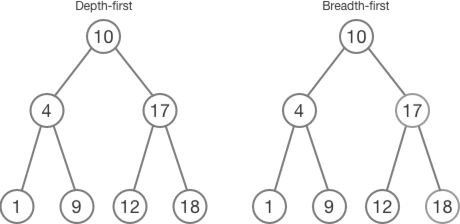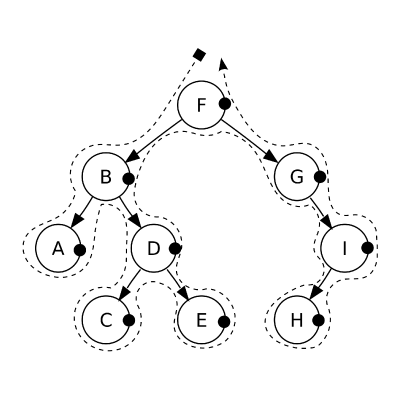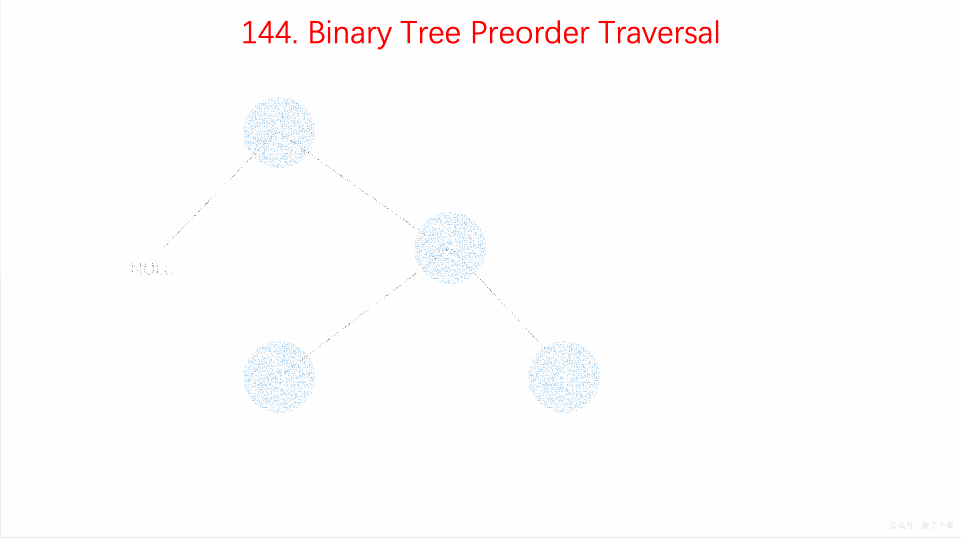# Iterative Preorder Traversal of Binary tree

## Introduction

In computer science, the traversal of a tree, also known as (walking to the tree), is a form of graph traversal that refers to the processing or visiting each node in a tree data structure exactly once.Source:- Giphy

Unlike linked lists, one-dimensional arrays or other linear data structures are traversed in a linear order. In contrast, trees can be traversed in various ways, including depth-first-order (pre-order, in-order, and post-order) or breadth-first-order (level-wise traversal). Below is an example of the DFS(depth-first-search- pre-order) and BFS(breadth-first-search):Source:- Medium

DFS, which stands for Depth-first search, is a depth-based technique in which the search tree starts traversing at the node and explores as far as feasible in terms of the depth of the tree, as shown in the above diagram. Thus, If we try to print the values, the output will be:

DFS : 10 4 1 9 17 12 18

BFS : 10 4 17 1 9 12 18

This article will look at how a binary tree can be Iteratively traversed using Preorder traversal, which resides under the DFS. In a depth-first traversal, you have three options: for example, root, left subtree, and right subtree. Preorder traversal is our emphasis; thus, we'll utilise examples of it. There are, however, several tree traversal algorithms based on the order in which you travel through these three i.e the order in which we visit the root, left subtree, and right subtree of the tree.

Now that you have seen the glimpse of the BFS vs DFS, let’s get started with today’s topic, the Iterative Preorder Traversal of Binary Trees.

## What is a Preorder Traversal?

As the name suggests, the root is visited first in preorder traversal, followed by the left and right subtreePreorder traversal can also be used to obtain a prefix expression from an expression tree.

Consider the below example to understand the Preorder Traversal:Source: Medium

Output = F B A D C E G I H

Explanation:- Suppose you are walking on a beach; the root node F is the first to be visited, followed by B, and then A. Now that there are no more left nodes to visit, you travel in the right direction, i.e., to the B node that has already been visited. Then you'll go to the right node D, then to its left node C, and finally, its right node E. The same procedure goes for the right subtree of the root node F

Great, now that we have learned what the preorder traversal is, let's traverse a binary tree using preorder and implement it in any programming language.

As we are performing the Iterative approach thus, we need an additional data structure to process each node in a binary tree. Hence, we’ll use the stack data structure, which follows the LIFO( Last-in-first-out) algorithm.

The algorithm will look like this:

## Algorithm

Step 1: Make an empty stack nodeStack and add the root node to it.

Step 2: Perform the following actions until the Stack becomes empty.

a) Pop and print an item from the stack.

b) Push the right child of a popped item to stack.

c) Push the left child of a popped item to stack.

To ensure that the left subtree is handled first, the right child is pushed before the left child as a stack is a LIFO data structure.

The above algorithm will look like this:Source:- Medium

In the preceding example, root node 1 is visited first. Therefore it is placed into the stack and popped out simultaneously, as stated in the prior algorithm. Because the root had no left child, the value was extracted from right subtree node and popped out simultaneously. Afterwards, the right node 4 of the right subtree is pushed before the left node 3 to ensure that the left subtree is handled first.

Thus, the result will be: 1 2 3 4

### Pseudo Code

Now, let’s see the implementation of the stated approach:

## Implementation in C++

Output

Time Complexity: O(n), where n is the total number of nodes in a tree as we are traversing the whole binary tree.

Space Complexity: O(h), where h is the height of the tree due to the space consumed in maintaining the stack. The height of the tree can be n for skewed binary trees, where n is the number of nodes in the binary tree.

As we can see, to perform the Iterative traversal, all nodes must be pushed onto the stack, which is not the most efficient method.

We can optimize the above method i.e only to push the right child nodes onto the stack. To clarify, the aim is to traverse the tree from the root node, printing the left child as long as it exists while concurrently pushing the right child of every node if it exists onto an auxiliary stack. Once we reach a null node, we pop the right child from the auxiliary stack, make our current node as this popped node and continue the above procedure until the stack is not empty.

### Pseudo Code

Below is the Implementation for the same:

## The Space-Optimized approach in C++

Output

Time Complexity:- O(n), where n is the total number of nodes in a tree as we are traversing the whole binary tree.

Space Complexity:- O(h), where h is the height of the tree due to the space consumed in maintaining the stack. The height of the tree can be n for skewed binary trees, where n is the number of nodes in the binary tree.

Now, you have understood the approaches, it’s time to implement the code on your own in any programming language on CodeStudio.

1. Is DFS and preorder traversal the same thing?
Preorder Traversal is another variant of DFS. Depth-first search (DFS) traverses the search tree as much as possible before proceeding to the next sibling, which is similar to pre-order traversal.

2. How deep is a Complete Binary Tree with n nodes?
Dn=log 2 (n+1) is the depth of a complete binary tree with n nodes. The depth of the tree is Dn, and the number of nodes is n. A complete binary tree is the one in which all levels, except possibly the last, have the maximum number of nodes.

3. What is an Iterative traversal?
Recursively traversing a binary tree is generally the initial way to tackle binary tree challenges. However, recursion may result in colossal memory footprints, and interviewers frequently request an iterative traverse. It is usual to utilise a stack or a queue while traversing a tree iteratively.

4. What is a binary tree, and what are its types?
A binary tree is a tree data structure in which each node has two offspring, referred to as the left and right children.

There are six types of binary trees:-

1. Full binary tree
2. Perfect binary tree
3. Complete binary tree
4. Degenerate or Pathological binary tree
5. Skewed binary tree
6. Balanced binary tree

## Key takeaways

To recapitulate, we covered the Preorder traversal of a binary tree utilising an iterative technique, in which we processed each node using the stack data structure. We discussed the iterative strategy, but there's another way to traverse a binary tree using recursive, which we'll discuss in our upcoming blogs. Until then, keep reading these articles to make your coding experience even more enjoyable.

Ninja, have fun learning!